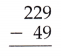Practice questions available in McGraw Hill Math Grade 6 Answer Key PDF Lesson 1.2 Adding and Subtracting Whole Numbers will engage students and is a great way of informal assessment.

Question 1.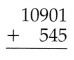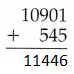By adding 10901 and 545 we get 11446

Question 2.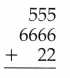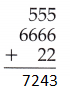By adding three numbers 555, 6666 and 22 we get 7243.

Question 3.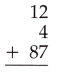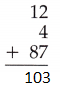By adding three numbers 12, 4 and 87 we get 103.

Question 4.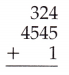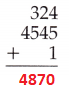By adding three numbers 324, 4545 and 1 we get 4870.

Question 5.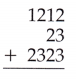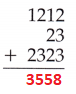By adding three numbers 1212, 23 and 2323 we get 3558.

Question 6.By adding three numbers 65, 10 and 2374 we get 2449.

Question 7.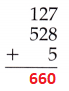By adding three numbers 127, 528 and 5 we get 660.

Question 8.By adding four given numbers 389, 28456, 21 and 2 we get 28868.

Question 9.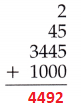By adding four given numbers 2, 45, 3445 and 1000 we get 4492.

Question 10.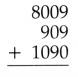By adding three numbers 8009, 909 and 1090 we get 10008.

Question 11.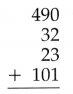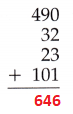By adding four given numbers 490, 32, 23 and 101 we get 646.

Question 12.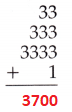By adding four given numbers 33, 333, 3333 and 1 we get 3700.

Question 13.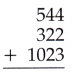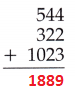By adding three numbers 544, 322 and 1023 we get 1889.

Question 14.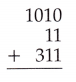By adding three numbers 1010, 11 and 311 we get 1332

Question 15.By adding three numbers 212, 355 and 22 we get 589.

Question 16.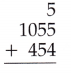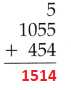By adding three numbers 5, 1055 and 454 we get 1514.

Question 17.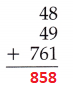By adding three numbers 48, 49 and 761 we get 858.

Question 18.By adding three numbers 34, 344 and 43 we get 421.

Question 19.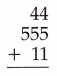By adding three numbers 44, 555 and 11 we get 610.

Question 20.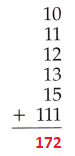Exercises Subtract

Question 1.By subtracting 7 from 22 we get 15.

Question 2.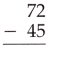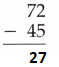By subtracting 45 from 72 we get 27.

Question 3.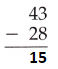By subtracting 28 from 43 we get 15.

Question 4.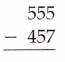By subtracting 457 from 555 we get 98.

Question 5.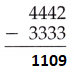By subtracting 3333 from 4442 we get 1109.

Question 6.By subtracting 999 from 2001 we get 1002.

Question 7.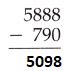By subtracting 790 from 5888 we get 5098.

Question 8.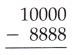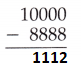By subtracting 8888 from 10000 we get 1112.

Question 9.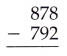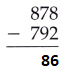By subtracting 792 from 878 we get 86.

Question 10.By subtracting 175 from 313 we get 138.

Question 11.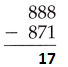By subtracting 871 from 888 we get 17.

Question 12.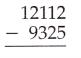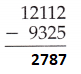By subtracting 9325 from 12112 we get 2787.

Question 13.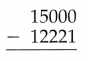By subtracting 12221 from 15000 we get 2779.

Question 14.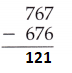By subtracting 676 from 767 we get 121.

Question 15.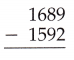By subtracting 1592 from 1689 we get 97.

Question 16.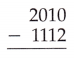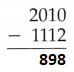By subtracting 1112 from 2010 we get 898.

Question 17.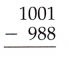By subtracting 988 from 1001 we get 13.

Question 18.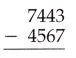Question 19.